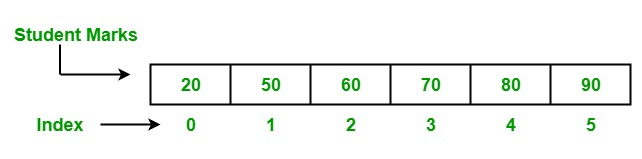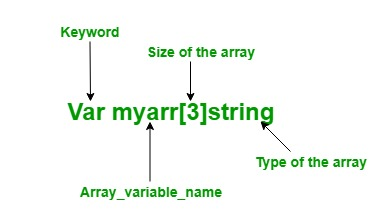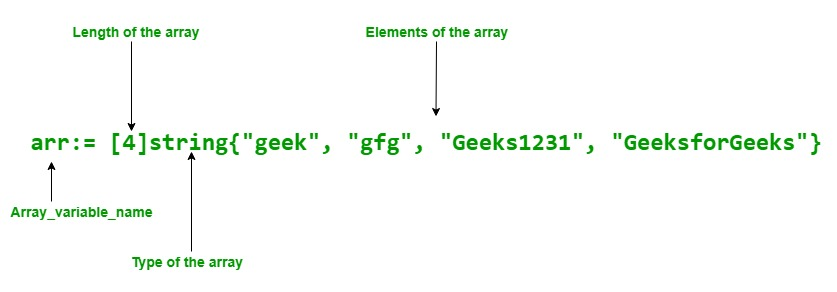GFG App
Open AppBrowser
Continue

Arrays in Golang or Go programming language is much similar to other programming languages. In the program, sometimes we need to store a collection of data of the same type, like a list of student marks. Such type of collection is stored in a program using an Array. An array is a fixed-length sequence that is used to store homogeneous elements in the memory. Due to their fixed length array are not much popular like Slice in Go language. In an array, you are allowed to store zero or more than zero elements in it. The elements of the array are indexed by using the [] index operator with their zero-based position, which means the index of the first element is array and the index of the last element is array[len(array)-1]### Creating and accessing an Array

In Go language, arrays are created in two different ways:

Using var keyword: In Go language, an array is created using the var keyword of a particular type with name, size, and elements. Syntax:

`Var array_name[length]Type`

Important Points:

In Go language, arrays are mutable, so that you can use array[index] syntax to the left-hand side of the assignment to set the elements of the array at the given index.

`Var array_name[index] = element`• You can access the elements of the array by using the index value or by using for loop.
• In Go language, the array type is one-dimensional.
• The length of the array is fixed and unchangeable.
• You are allowed to store duplicate elements in an array.

Approach 1: Using shorthand declaration:

In Go language, arrays can also declare using shorthand declaration. It is more flexible than the above declaration.

Syntax:

`array_name:= [length]Type{item1, item2, item3,...itemN}`Example:

## Go

 `// Go program to illustrate how to create ` `// an array using shorthand declaration  ` `// and accessing the elements of the  ` `// array using for loop ` `package` `main ` ` `  `import` `"fmt"` ` `  `func` `main() { ` ` `  `// Shorthand declaration of array ` `arr:= [``4``]``string``{``"geek"``, ``"gfg"``, ``"Geeks1231"``, ``"GeeksforGeeks"``} ` ` `  `// Accessing the elements of  ` `// the array Using for loop ` `fmt.Println(``"Elements of the array:"``) ` ` `  `for` `i:= ``0``; i < ``3``; i++{ ` `fmt.Println(arr[i]) ` `} ` ` `  `} `

Output:

```Elements of the array:
geek
gfg
Geeks1231```

### Multi-Dimensional Array

As we already know that arrays are 1-D but you are allowed to create a multi-dimensional array. Multi-Dimensional arrays are the arrays of arrays of the same type. In Go language, you can create a multi-dimensional array using the following syntax:

`Array_name[Length1][Length2]..[LengthN]Type`

You can create a multidimensional array using Var keyword or using shorthand declaration as shown in the below example.

Note: In a multi-dimension array, if a cell is not initialized with some value by the user, then it will initialize with zero by the compiler automatically. There is no uninitialized concept in the Golang.

Example:

## Go

 `// Go program to illustrate the ` `// concept of multi-dimension array ` `package` `main ` ` `  `import` `"fmt"` ` `  `func` `main() { ` ` `  `    ``// Creating and initializing ` `    ``// 2-dimensional array ` `    ``// Using shorthand declaration ` `    ``// Here the (,) Comma is necessary ` `    ``arr := [``3``][``3``]``string``{{``"C #"``, ``"C"``, ``"Python"``}, {``"Java"``, ``"Scala"``, ``"Perl"``}, ` `        ``{``"C++"``, ``"Go"``, ``"HTML"``}} ` ` `  `    ``// Accessing the values of the ` `    ``// array Using for loop ` `    ``fmt.Println(``"Elements of Array 1"``) ` `    ``for` `x := ``0``; x < ``3``; x++ { ` `        ``for` `y := ``0``; y < ``3``; y++ { ` ` `  `            ``fmt.Println(arr[x][y]) ` `        ``} ` `    ``} ` ` `  `    ``// Creating a 2-dimensional ` `    ``// array using var keyword ` `    ``// and initializing a multi ` `    ``// -dimensional array using index ` `    ``var` `arr1 [``2``][``2``]int ` `    ``arr1[``0``][``0``] = ``100` `    ``arr1[``0``][``1``] = ``200` `    ``arr1[``1``][``0``] = ``300` `    ``arr1[``1``][``1``] = ``400` ` `  `    ``// Accessing the values of the array ` `    ``fmt.Println(``"Elements of array 2"``) ` `    ``for` `p := ``0``; p < ``2``; p++ { ` `        ``for` `q := ``0``; q < ``2``; q++ { ` `            ``fmt.Println(arr1[p][q]) ` `        ``} ` `    ``} ` `} `

Output:

```Elements of Array 1
C#
C
Python
Java
Scala
Perl
C++
Go
HTML
Elements of array 2
100
200
300
400```

In an array, if an array does not initialize explicitly, then the default value of this array is 0

Example:

## Go

 `// Go program to illustrate an array ` `package` `main ` ` `  `    ``import` `"fmt"` ` `  `func` `main() ` `{ ` ` `  `    ``// Creating an array of int type ` `    ``// which stores, two elements ` `    ``// Here, we do not initialize the ` `    ``// array so the value of the array ` `    ``// is zero ` `    ``var` `myarr[``2``] int fmt.Println(``"Elements of the Array: "``, myarr) ` `}`

Output:

`Elements of the Array : [0 0]`

In an array, you can find the length of the array using len() method as shown below:

Example:

## Go

 `// Go program to illustrate how to find ` `// the length of the array ` `package` `main ` ` `  `import` `"fmt"` ` `  `func` `main() { ` ` `  `// Creating array ` `// Using shorthand declaration     ` `arr1:= [``3``]int{``9``,``7``,``6``} ` `arr2:= [...]int{``9``,``7``,``6``,``4``,``5``,``3``,``2``,``4``} ` `arr3:= [``3``]int{``9``,``3``,``5``} ` ` `  `// Finding the length of the  ` `// array using len method ` `fmt.Println(``"Length of the array 1 is:"``, len(arr1)) ` `fmt.Println(``"Length of the array 2 is:"``, len(arr2)) ` `fmt.Println(``"Length of the array 3 is:"``, len(arr3)) ` `} `

Output:

```Length of the array 1 is: 3
Length of the array 2 is: 8
Length of the array 3 is: 3```

In an array, if ellipsis ‘‘…’’ become visible at the place of length, then the length of the array is determined by the initialized elements. As shown in the below example:

Example:

## Go

 `// Go program to illustrate the ` `// concept of ellipsis in an array ` `package` `main ` ` `  `import` `"fmt"` ` `  `func` `main() { ` `     `  `// Creating an array whose size is determined  ` `// by the number of elements present in it ` `// Using ellipsis ` `myarray:= [...]``string``{``"GFG"``, ``"gfg"``, ``"geeks"``, ` `                    ``"GeeksforGeeks"``, ``"GEEK"``} ` ` `  `fmt.Println(``"Elements of the array: "``, myarray) ` ` `  `// Length of the array ` `// is determine by  ` `// Using len() method ` `fmt.Println(``"Length of the array is:"``, len(myarray)) ` `} `

Output:

```Elements of the array:  [GFG gfg geeks GeeksforGeeks GEEK]
Length of the array is: 5```

In an array, you are allowed to iterate over the range of the elements of the array. As shown in the below example:

Example:

## Go

 `// Go program to illustrate  ` `// how to iterate the array ` `package` `main ` ` `  `import` `"fmt"` ` `  `func` `main() { ` `     `  `// Creating an array whose size ` `// is represented by the ellipsis ` `myarray:= [...]int{``29``, ``79``, ``49``, ``39``, ` `                   ``20``, ``49``, ``48``, ``49``} ` ` `  `// Iterate array using for loop ` `for` `x:=``0``; x < len(myarray); x++{ ` `fmt.Printf(``"%d\n"``, myarray[x]) ` `} ` `} `

Output:

```29
79
49
39
20
49
48
49```

In Go language, an array is of value type not of reference type. So when the array is assigned to a new variable, then the changes made in the new variable do not affect the original array. As shown in the below example: Example:

## Go

 `// Go program to illustrate value type array ` `package` `main ` ` `  `import` `"fmt"` ` `  `func` `main() { ` `     `  `// Creating an array whose size  ` `// is represented by the ellipsis ` `my_array:= [...]int{``100``, ``200``, ``300``, ``400``, ``500``} ` `fmt.Println(``"Original array(Before):"``, my_array) ` ` `  `// Creating a new variable  ` `// and initialize with my_array ` `new_array := my_array ` ` `  `fmt.Println(``"New array(before):"``, new_array) ` ` `  `// Change the value at index 0 to 500 ` `new_array[``0``] = ``500` ` `  `fmt.Println(``"New array(After):"``, new_array) ` ` `  `fmt.Println(``"Original array(After):"``, my_array) ` `} `

Output:

```Original array(Before): [100 200 300 400 500]
New array(before): [100 200 300 400 500]
New array(After): [500 200 300 400 500]
Original array(After): [100 200 300 400 500]```

In an array, if the element type of the array is comparable, then the array type is also comparable. So we can directly compare two arrays using == operator. As shown in the below example:

Example:

## Go

 `// Go program to illustrate  ` `// how to compare two arrays ` `package` `main ` ` `  `import` `"fmt"` ` `  `func` `main() { ` ` `  `// Arrays     ` `arr1:= [``3``]int{``9``,``7``,``6``} ` `arr2:= [...]int{``9``,``7``,``6``} ` `arr3:= [``3``]int{``9``,``5``,``3``} ` ` `  `// Comparing arrays using == operator ` `fmt.Println(arr1==arr2) ` `fmt.Println(arr2==arr3) ` `fmt.Println(arr1==arr3) ` ` `  `// This will give and error because the  ` `// type of arr1 and arr4 is a mismatch  ` `/* ` `arr4:= int{9,7,6} ` `fmt.Println(arr1==arr4) ` `*/` `} `

Output:

```true
false
false```

My Personal Notes arrow_drop_up